# Geometric PDEs in Freiburg 2018

## Speakers

•### Tuesday 9h00: Concentration sets for multiple equal-depth wells potentials in the 2D elliptic case

The formation of codimension-one interfaces for multiwell gradient-driven problems is well-known and established in the scalar case, where the equation is often referred to as the Allen-Cahn equation. The vectorial case in contrast is quite open. This lack of results and insight is to a large extend related to the absence of known appropriate monotonicity formula. I will focus on the elliptic case in two dimensions, and describe some methods which allow to circumvent the lack of monotonicity formula.

•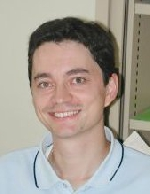### Tuesday 10h30: Density of bounded maps in Sobolev spaces into closed manifolds

A minimizing harmonic map u from the unit ball B in R^m with values into a manifold N is smooth outside a small singular set. Some of these singularitites are topologically non trivial, in the sense that u cannot be approximated in a neighborhood of this singular set by smooth maps with values into N. In this talk, we present classical and more recent counterexamples and positive results on the density of smooth maps C^{infty}(overline{B};N) in Sobolev spaces W^{1,p}(B;N), both for the strong and the weak topology. We consider the case when the target manifold N is closed but not necessarily compact, where both the geometry and the topology of N play a crucial role. This is a joint work with Augusto Ponce and Jean Van Schaftingen.

•### Tuesday 15h00: Distance comparison for Curve Shortening of Networks

The curve shortening flow for networks, first introduced by Mullins to study the evolution of grain boundaries models two-dimensional multiphase systems capturing the dynamics of interface boundaries. It is also an example of a Brakke fow by Mean Curvature, that is necessarily singular, exhibiting qualitative differences in behaviour to the smooth case. This talk will discuss joint work in progress with Mariel Sáez adapting distance comparison techniques previously used in the smooth setting to non-smooth regimes. Many novel features arise such as non-uniqueness of embedded, self-similar solutions, and generally expected singular behaviour. Understanding singularity formation is an actively studied topic and is an essential ingredient for further analysis and global results for the flow. The distance comparison techniques described in this talk may prove useful in such analysis.

•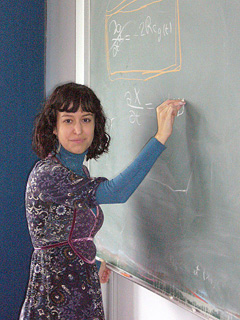### Monday 10h30: Brownian motion on Perelman's almost Ricci-flat manifold

We study Brownian motion and stochastic parallel transport on Perelman's almost Ricci flat manifold, whose dimension depends on a parameter \$N\$ unbounded from above. By taking suitable projections we construct sequences of space-time Brownian motion and stochastic parallel transport whose limit as N -> infty are the corresponding objects for the Ricci flow. In order to make precise this process of passing to the limit, we study the martingale problems for the Laplace operator on Perelman’s manifold and for the horizontal Laplacian on the corresponding orthonormal frame bundle. As an application, we see how the characterizations of two-sided bounds on the Ricci curvature established by A. Naber applied to Perelman's manifold lead to the inequalities that characterize solutions of the Ricci flow discovered by Naber and Haslhofer. This is joint work with Robert Haslhofer.

### Tuesday 16:30: Existence for Dirac equations and Dirac-harmonic maps

Dirac-harmonic maps are solutions of a coupled system of a Dirac equation and harmonic map type equation. They are critical points of a functional coming from the nonlinear supersymmetric sigma model, generalizing both the classical harmonic maps and harmonic spinors. However, the general existence problem for them turn out to be challenging. In this talk, we will discuss the existence and uniqueness of solutions of Dirac equations satisfying a class of local elliptic boundary value conditions, and the existence of Dirac-harmonic maps and their heat flows. This is based on joint works with J.Jost, J.Y.Li, L.L.Sun, G.F.Wang and M.M.Zhu respectively.

•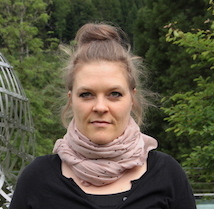### Wednesday 16h00: Area-preserving curve shortening flow

Under the enclosed area-preserving curve shortening flow arbitrary non-convex embedded curves do not necessary stay embedded. But initial curves with the sharp lower lower bound of -pi for the angles between tangent vectors stay embedded and stay smooth for all times. Type-I singularities in finite time can be excluded by a monotonicity formula. Furthermore, a distance comparison principle excludes type-II singularities in finite time. Once a flow exists for all times, the curves become convex in finite time and converge to a round circle.

•### Wednesday 16h30: Analytic remarks on 2-gauge theory

2-Gauge theory is a theory from mathematical physics that roughly has the same scaling and invariance properties on 6-manifolds that usual gauge theory has on 4-manifolds. There is a sort of 2-Yang-Mills-functional that locally controls Sobolev norms of 2-connections whenever they are in a 'good gauge'. We discuss the problem of finding a good gauge and show that the answer is simpler than in classical gauge theory.

### (room: Rundbau) Monday 15h00: Symmetric Critical Points of the Integral Menger Curvature

In geometric knot theory, one tries to examine knots by using energy functionals. These so-called knot energies shall determine whether and to what extent a curve is knotted. We focus on the integral Menger curvature and try to evaluate its energy landscape. Using a modified version of Palais' symmetric criticality principle, we show the existence of at least two distinct critical points in torus knot classes. Furthermore, we apply a numerical gradient flow to approximate energy values of the symmetric critical points and the minimiser in the whole knot class. In addition, we gain numerical evidence concerning the nature of the newly gained critical points.

### Tuesday 11h30: On higher dimensional singularities for the fractional Yamabe problem: a non-local Mazzeo-Pacard program

We consider the problem of constructing solutions to the fractional Yamabe problem that are singular at a given smooth sub-manifold, for which we establish the classical gluing method of Mazzeo and Pacard for the scalar curvature in the fractional setting. This proof is based on the analysis of the model linearized operator, which amounts to the study of a fractional order ODE, and thus our main contribution here is the development of new methods coming from conformal geometry and scattering theory for the study of non-local ODEs. Note, however, that no traditional phase-plane analysis is available here. Instead, first, we provide a rigorous construction of radial fast-decaying solutions by a blow-up argument and a bifurcation method. Second, we use conformal geometry to rewrite this non-local ODE, giving a hint of what a non-local phase-plane analysis should be. Third, for the linear theory, we use complex analysis and some non-Euclidean harmonic analysis to examine a fractional Schrödinger equation with a Hardy type critical potential. We construct its Green's function, deduce Fredholm properties, and analyze its asymptotics at the singular points in the spirit of Frobenius method. Surprisingly enough, a fractional linear ODE may still have a two-dimensional kernel as in the second order case.

•### Friday 11:30: Interior \$C^2\$ estimates for immersed hypersurfaces in \$R^{n+1}\$.

Heintz proved in 1960s the interior \$C^2\$ estimate for immersed surfaces with positive Gauss curvature in \$R^3\$. The result is a consequence of his interior estimate for Monge-Ampere equation in dimension 2. Such interior estimate is false if \$n>2\$ by counter-examples of Pogorelov. It is also false for convex solutions of k-Hessian equations and for k-mean curvature equations when K>2 in general. It has been a longstanding problem of the question of interior \$C^2\$ estimates for solutions of 2-Hessian equation and for prescribing scalar curvature equations. We will present recent results jointly with Guohuan Qiu, providing an affirmative answer for convex solutions of these equations. The argument yields interior \$C^2\$ estimate for immersed hypersurfaces with positive intrinsic scalar curvature in any dimension.

### Tuesday 14h00: Estimates for some fully nonlinear elliptic equations on manifolds

Establishing a priori estimates is crucial in study of nonlinear PDEs. In this talk we discuss some recent progresses in this direction. We shall consider some fully nonlinear equations in connection with conformal deformation of Chen-Ricci curvatures on Hermintian manifolds, a Loewner-Nirenberg type theorem, as well as the Neumann problem for Hessian equations on Riemannian manifolds, if time allows.

•### Wednesday 09h00: Inverse mean curvature flow for entire graphs

The lecture describes joint work with P. Daskalopoulos on regularity estimates and long-time existence results for solutions of inverse mean curvature flow, that can be represented as entire graphs. In particular, we show a general existence result for mean convex graphs of superlinear growth and then treat convex graphs in the critical case of linear growth.

•### Tuesday 16h00: Higher codimensional spacelike mean curvature flow and applications

We consider entire higher codimensional mean curvature flow in \$R^{n,m}\$ of \$n\$-dimensional spacelike manifolds and prove a long time existence theorem starting from arbitrary spacelike initial data. If there is time we will discuss applications of these results to the \$G_2\$-Laplacian flow.

### Thursday 10h30: John-Nirenberg Inequality and Collapse in Conformal Geometry

Let \$g\$ be a metric over \$B\$, and \$g_k=u_k^frac{4}{n-2}g\$. We assume \$||R(g_k)||_{L^p}<C\$, where \$R\$ is the scalar curvature and \$p>frac{n}{2}\$. We will use John-Nirenberg inequality to prove that if \$vol(B,g_k) rightarrow 0\$, then there exists \$c_k rightarrow + infty\$, such that \$c_k u_k\$ converges to a positive function weakly in \$W^{2,p}_{loc}(B)\$. As an application, we will study the bubble tree convergence of a conformal metric sequence with integral-bounded scalar curvature.

•### Wednesday 15h00: Boundary regularity of minimizing biharmonic maps

Biharmonic maps (i.e., critical points of the Hessian energy) can be seen as a generalization of harmonic maps into 4th order problems. It is known that (even minimizing) biharmonic maps may have singularities. We prove that, for sufficiently smooth boundary data minimizing biharmonic maps must be smooth in a neighborhood of the boundary.

•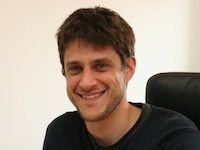### (room: Rundbau) Monday 16:30: Fractional harmonic maps & local/nonlocal minimal surfaces

In this talk, I will report on some recent regularity results for fractional harmonic maps into a manifold. The equation for this kind of maps is the fractional analogue of the classical harmonic map system where the Laplacian is replaced by a fractional Laplacian as defined in Fourier space. I will explain the relations between fractional harmonic maps and local or nonlocal minimal surfaces depending on the fractional exponent, and how this relations lead to an improved regularity for sphere targets.

•### (room: Rundbau) Monday 16h00: Singularities of minimizing harmonic maps

Minimizing harmonic maps between manifolds are known to be smooth outside the so-called singular set. In general this is a rectifable set of codimension 3, i.e., it can be covered by countably many Lipschitz pieces, but still may have many small gaps. In one special case of maps from a 4-dimensional domain into the 2-dimensional sphere Hardt and Lin proved that the singular set consists of topological curves. I will show a generalization to higher dimensional domains and discuss the topological obstruction responsible for preventing gaps in the singular set.

•### Thursday 11h30: Hyperbolic solutions to Bernoulli's free boundary problem

Bernoulli's free boundary problem is an overdetermined problem in which one seeks an annular domain such that the capacitary potential satisfies an extra boundary condition. There exist two different types of solutions: elliptic and hyperbolic solutions. Elliptic solutions are ``stable'' solutions and tractable by variational methods and maximum principles, while hyperbolic solutions are ``unstable'' solutions of which the qualitative behavior is less known. I will present a recent joint work with Antoine Henrot in which we show the existence and qualitative behavior of hyperbolic solutions by a new flow approach.

### Monday 11h30: Noncompact Stability

We discuss stability questions for noncompact solutions to Ricci and mean curvature flow.

•### Wednesday 10h30: Optimal isoperimetric inequalities for 2-dimensional surfaces in Hadamard-Cartan manifolds in any codimension

Let (M^n,g) be simply connected, complete, with non-positive sectional curvatures, and Sigma a 2-dimensional closed integral current (or flat chain mod 2) with compact support in M. Let S be an area minimising integral 3-current (resp. flat chain mod 2) such that partial S = Sigma. We use a weak mean curvature flow, obtained via elliptic regularisation, starting from Sigma, to show that S satisfies the optimal Euclidean isoperimetric inequality: 6 sqrt{pi} M[S] leq (M[Sigma])^{3/2}. We also obtain an optimal estimate in case the sectional curvatures of M are bounded from above by -kappa < 0 and characterise the case of equality. The proof follows from an almost monotonicity of a suitable isoperimetric difference along the approximating flows in one dimension higher and an optimal estimate for the Willmore energy of a 2-dimensional integral varifold with first variation summable in L^2

•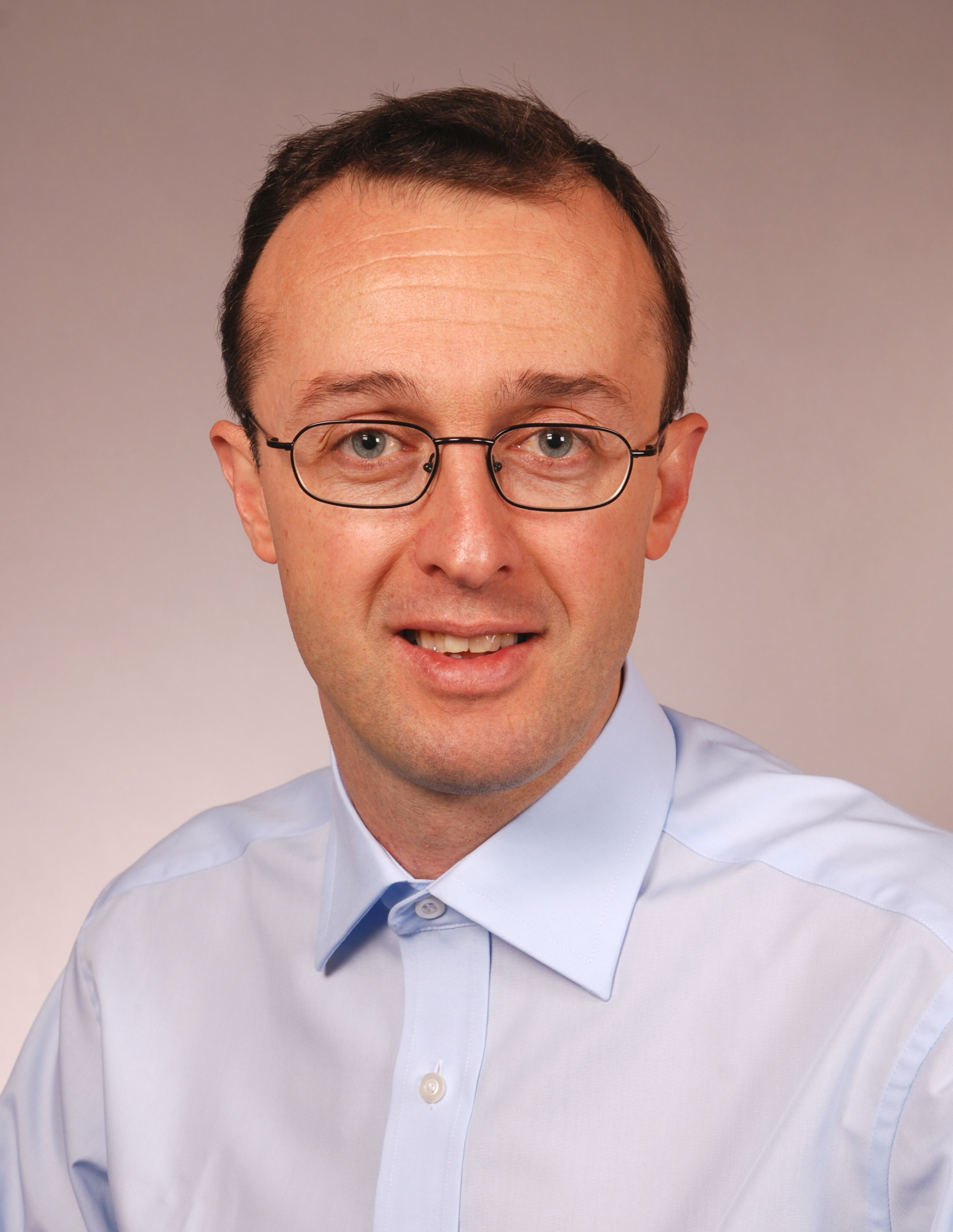### Wednesday 11h30: Integral estimates for Ricci flow in four dimensions

We prove some integral estimates for Ricci flows on compact manifolds in four dimensions which hold for any solution. We explain some consequences of these estimates if one further assumes that the scalar curvature is bounded up to the singular time.

•### Friday 10h30: Mean curvature flow of Whitney spheres

It is shown that an equivariant Lagrangian sphere with a positivity condition on its Ricci curvature develops a type-II singularity under the Lagrangian mean curvature flow that rescales to the product of a grim reaper with a flat Lagrangian subspace. In particular this result applies to the Whitney spheres.

•### Friday 09h00: Pyramid Ricci flows

I will discuss some recent theory for starting Ricci flows in situations where no classical solution is expected to exist. My plan is to emphasise the (geometric) PDE theory rather than the applications, with the so-called Pyramid Extension Lemma a key target. Joint work with Miles Simon and Andrew McLeod.

•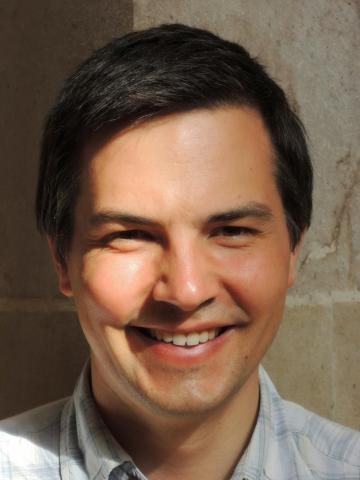### (room: Rundbau) Monday 14h00: Uniform boundedness principle for Sobolev maps between manifolds

The classical uniform boundedness principle of Banach and Steinhaus for linear operators between Banach spaces is a theorem that transforms a bound depending on the point in the domain into a global uniform bound. The nonlinear character of Sobolev spaces between manifolds makes it unapplicable in these spaces. By relying on the structure of the domain of Sobolev maps, we have obtained a quite general uniform boundedness principle for energies of Sobolev maps, which allows us to recover known estimates and counterexamples for the problems of weak-bounded approximation, of extension of traces, of lifting and of superposition. The result covers fractional and first order Sobolev spaces.

•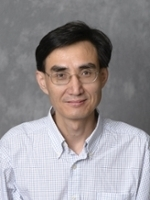### Wednesday 14h00: Some recent results on mathematical analysis of Ericksen-Leslie System

The Ericksen-Leslie system is the governing equation that describes the hydrodynamic evolution of nematic liquid crystal materials, first introduced by J. Ericksen and F. Leslie back in 1960's. It is a coupling system between the underlying fluid velocity field and the macroscopic average orientation field of the nematic liquid crystal molecules. Mathematically, this system couples the Navier-Stokes equation and the harmonic heat flow into the unit sphere. It is very challenging to analyze such a system by establishing the existence, uniqueness, and (partial) regularity of global (weak/large) solutions, with many basic questions to be further exploited. In this talk, I will discuss some results we have obtained from the last few years.

•### Thursday 9h00: Exterior Bernstein and Bernstein problems for Hessian equations

We survey some new and old uniqueness results for Hessian equations such as special Lagrangian equations, Monge-Ampere equations, and symmetric Hessian equations. In particular, a unified approach to quadratic asymptote of solutions over exterior domains--based on an 'exterior' Evans-Krylov, corresponding to Allard-Almgren's uniqueness of tangent cones in minimal surface situation -- will be presented (joint with D.S. Li and Zh.S. Li). Special Lagrangian and Monge-Ampere equations are the potential equations for minimal and maximal 'gradient' graphs in Euclid and pseudo-Euclid spaces respectively.

## Schedule

Time Speaker Description

# Monday (July 23rd, 2018)

Monday 10h00 Registration
Monday 10h30Esther Cabezas-Rivas: Brownian motion on Perelman's almost Ricci-flat manifold Frankfurt University We study Brownian motion and stochastic parallel transport on Perelman's almost Ricci flat manifold, whose dimension depends on a parameter \$N\$ unbounded from above. By taking suitable projections we construct sequences of space-time Brownian motion and stochastic parallel transport whose limit as N -> infty are the corresponding objects for the Ricci flow. In order to make precise this process of passing to the limit, we study the martingale problems for the Laplace operator on Perelman’s manifold and for the horizontal Laplacian on the corresponding orthonormal frame bundle. As an application, we see how the characterizations of two-sided bounds on the Ricci curvature established by A. Naber applied to Perelman's manifold lead to the inequalities that characterize solutions of the Ricci flow discovered by Naber and Haslhofer. This is joint work with Robert Haslhofer.
Monday 11h30 Oliver Schnürer: Noncompact Stability University of Konstanz We discuss stability questions for noncompact solutions to Ricci and mean curvature flow.
Monday 12h30 Lunch
Monday 14h00(room: Rundbau) Jean Van Schaftingen: Uniform boundedness principle for Sobolev maps between manifolds UC Louvain The classical uniform boundedness principle of Banach and Steinhaus for linear operators between Banach spaces is a theorem that transforms a bound depending on the point in the domain into a global uniform bound. The nonlinear character of Sobolev spaces between manifolds makes it unapplicable in these spaces. By relying on the structure of the domain of Sobolev maps, we have obtained a quite general uniform boundedness principle for energies of Sobolev maps, which allows us to recover known estimates and counterexamples for the problems of weak-bounded approximation, of extension of traces, of lifting and of superposition. The result covers fractional and first order Sobolev spaces.
Monday 15h00 (room: Rundbau) Alexandra Gilsbach: Symmetric Critical Points of the Integral Menger Curvature RWTH Aachen In geometric knot theory, one tries to examine knots by using energy functionals. These so-called knot energies shall determine whether and to what extent a curve is knotted. We focus on the integral Menger curvature and try to evaluate its energy landscape. Using a modified version of Palais' symmetric criticality principle, we show the existence of at least two distinct critical points in torus knot classes. Furthermore, we apply a numerical gradient flow to approximate energy values of the symmetric critical points and the minimiser in the whole knot class. In addition, we gain numerical evidence concerning the nature of the newly gained critical points.
Monday 15h30 Coffeee Break
Monday 16h00(room: Rundbau) Michał Miśkiewicz: Singularities of minimizing harmonic maps University of Warsaw Minimizing harmonic maps between manifolds are known to be smooth outside the so-called singular set. In general this is a rectifable set of codimension 3, i.e., it can be covered by countably many Lipschitz pieces, but still may have many small gaps. In one special case of maps from a 4-dimensional domain into the 2-dimensional sphere Hardt and Lin proved that the singular set consists of topological curves. I will show a generalization to higher dimensional domains and discuss the topological obstruction responsible for preventing gaps in the singular set.
Monday 16:30(room: Rundbau) Vincent Millot: Fractional harmonic maps & local/nonlocal minimal surfaces Université Paris Diderot In this talk, I will report on some recent regularity results for fractional harmonic maps into a manifold. The equation for this kind of maps is the fractional analogue of the classical harmonic map system where the Laplacian is replaced by a fractional Laplacian as defined in Fourier space. I will explain the relations between fractional harmonic maps and local or nonlocal minimal surfaces depending on the fractional exponent, and how this relations lead to an improved regularity for sphere targets.

# Tuesday (July 24th, 2018)

Tuesday 9h00Fabrice Bethuel: Concentration sets for multiple equal-depth wells potentials in the 2D elliptic case Paris 6 The formation of codimension-one interfaces for multiwell gradient-driven problems is well-known and established in the scalar case, where the equation is often referred to as the Allen-Cahn equation. The vectorial case in contrast is quite open. This lack of results and insight is to a large extend related to the absence of known appropriate monotonicity formula. I will focus on the elliptic case in two dimensions, and describe some methods which allow to circumvent the lack of monotonicity formula.
Tuesday 10h00 Coffee break
Tuesday 10h30Pierre Bousquet: Density of bounded maps in Sobolev spaces into closed manifolds Université de Toulouse A minimizing harmonic map u from the unit ball B in R^m with values into a manifold N is smooth outside a small singular set. Some of these singularitites are topologically non trivial, in the sense that u cannot be approximated in a neighborhood of this singular set by smooth maps with values into N. In this talk, we present classical and more recent counterexamples and positive results on the density of smooth maps C^{infty}(overline{B};N) in Sobolev spaces W^{1,p}(B;N), both for the strong and the weak topology. We consider the case when the target manifold N is closed but not necessarily compact, where both the geometry and the topology of N play a crucial role. This is a joint work with Augusto Ponce and Jean Van Schaftingen."
Tuesday 11h30 Maria del Mar González Nogueras: On higher dimensional singularities for the fractional Yamabe problem: a non-local Mazzeo-Pacard program Universidad Autónoma de Madrid We consider the problem of constructing solutions to the fractional Yamabe problem that are singular at a given smooth sub-manifold, for which we establish the classical gluing method of Mazzeo and Pacard for the scalar curvature in the fractional setting. This proof is based on the analysis of the model linearized operator, which amounts to the study of a fractional order ODE, and thus our main contribution here is the development of new methods coming from conformal geometry and scattering theory for the study of non-local ODEs. Note, however, that no traditional phase-plane analysis is available here. Instead, first, we provide a rigorous construction of radial fast-decaying solutions by a blow-up argument and a bifurcation method. Second, we use conformal geometry to rewrite this non-local ODE, giving a hint of what a non-local phase-plane analysis should be. Third, for the linear theory, we use complex analysis and some non-Euclidean harmonic analysis to examine a fractional Schrödinger equation with a Hardy type critical potential. We construct its Green's function, deduce Fredholm properties, and analyze its asymptotics at the singular points in the spirit of Frobenius method. Surprisingly enough, a fractional linear ODE may still have a two-dimensional kernel as in the second order case.
Tuesday 12h30 Lunch
Tuesday 14h00 Bo Guan: Estimates for some fully nonlinear elliptic equations on manifolds Ohio State Establishing a priori estimates is crucial in study of nonlinear PDEs. In this talk we discuss some recent progresses in this direction. We shall consider some fully nonlinear equations in connection with conformal deformation of Chen-Ricci curvatures on Hermintian manifolds, a Loewner-Nirenberg type theorem, as well as the Neumann problem for Hessian equations on Riemannian manifolds, if time allows.
Tuesday 15h00Paul Bryan: Distance comparison for Curve Shortening of Networks Macquarie University The curve shortening flow for networks, first introduced by Mullins to study the evolution of grain boundaries models two-dimensional multiphase systems capturing the dynamics of interface boundaries. It is also an example of a Brakke fow by Mean Curvature, that is necessarily singular, exhibiting qualitative differences in behaviour to the smooth case. This talk will discuss joint work in progress with Mariel Sáez adapting distance comparison techniques previously used in the smooth setting to non-smooth regimes. Many novel features arise such as non-uniqueness of embedded, self-similar solutions, and generally expected singular behaviour. Understanding singularity formation is an actively studied topic and is an essential ingredient for further analysis and global results for the flow. The distance comparison techniques described in this talk may prove useful in such analysis.
Tuesday 15h30 Coffeee Break
Tuesday 16h00Ben Lambert: Higher codimensional spacelike mean curvature flow and applications University College London We consider entire higher codimensional mean curvature flow in \$R^{n,m}\$ of \$n\$-dimensional spacelike manifolds and prove a long time existence theorem starting from arbitrary spacelike initial data. If there is time we will discuss applications of these results to the \$G_2\$-Laplacian flow.
Tuesday 16:30 Qun Chen: Existence for Dirac equations and Dirac-harmonic maps Wuhan University Dirac-harmonic maps are solutions of a coupled system of a Dirac equation and harmonic map type equation. They are critical points of a functional coming from the nonlinear supersymmetric sigma model, generalizing both the classical harmonic maps and harmonic spinors. However, the general existence problem for them turn out to be challenging. In this talk, we will discuss the existence and uniqueness of solutions of Dirac equations satisfying a class of local elliptic boundary value conditions, and the existence of Dirac-harmonic maps and their heat flows. This is based on joint works with J.Jost, J.Y.Li, L.L.Sun, G.F.Wang and M.M.Zhu respectively.

# Wednesday (July 25th, 2018)

Wednesday 09h00Gerhard Huisken: Inverse mean curvature flow for entire graphs Oberwolfach The lecture describes joint work with P. Daskalopoulos on regularity estimates and long-time existence results for solutions of inverse mean curvature flow, that can be represented as entire graphs. In particular, we show a general existence result for mean convex graphs of superlinear growth and then treat convex graphs in the critical case of linear growth.
Wednesday 10h00 Coffee break
Wednesday 10h30Felix Schulze: Optimal isoperimetric inequalities for 2-dimensional surfaces in Hadamard-Cartan manifolds in any codimension University College London Let (M^n,g) be simply connected, complete, with non-positive sectional curvatures, and Sigma a 2-dimensional closed integral current (or flat chain mod 2) with compact support in M. Let S be an area minimising integral 3-current (resp. flat chain mod 2) such that partial S = Sigma. We use a weak mean curvature flow, obtained via elliptic regularisation, starting from Sigma, to show that S satisfies the optimal Euclidean isoperimetric inequality: 6 sqrt{pi} M[S] leq (M[Sigma])^{3/2}. We also obtain an optimal estimate in case the sectional curvatures of M are bounded from above by -kappa < 0 and characterise the case of equality. The proof follows from an almost monotonicity of a suitable isoperimetric difference along the approximating flows in one dimension higher and an optimal estimate for the Willmore energy of a 2-dimensional integral varifold with first variation summable in L^2.
Wednesday 11h30Miles Simon: Integral estimates for Ricci flow in four dimensions Universität Magdeburg We prove some integral estimates for Ricci flows on compact manifolds in four dimensions which hold for any solution. We explain some consequences of these estimates if one further assumes that the scalar curvature is bounded up to the singular time.
Wednesday 12h30 Lunch
Wednesday 14h00Changyou Wang: Some recent results on mathematical analysis of Ericksen-Leslie System Purdue University The Ericksen-Leslie system is the governing equation that describes the hydrodynamic evolution of nematic liquid crystal materials, first introduced by J. Ericksen and F. Leslie back in 1960's. It is a coupling system between the underlying fluid velocity field and the macroscopic average orientation field of the nematic liquid crystal molecules. Mathematically, this system couples the Navier-Stokes equation and the harmonic heat flow into the unit sphere. It is very challenging to analyze such a system by establishing the existence, uniqueness, and (partial) regularity of global (weak/large) solutions, with many basic questions to be further exploited. In this talk, I will discuss some results we have obtained from the last few years.
Wednesday 15h00Katarzyna Mazowiecka: Boundary regularity of minimizing biharmonic maps Université catholique de Louvain Biharmonic maps (i.e., critical points of the Hessian energy) can be seen as a generalization of harmonic maps into 4th order problems. It is known that (even minimizing) biharmonic maps may have singularities. We prove that, for sufficiently smooth boundary data minimizing biharmonic maps must be smooth in a neighborhood of the boundary.
Wednesday 15h30 Coffeee Break
Wednesday 16h00Friederike Dittberner: Area-preserving curve shortening flow Universität Konstanz Under the enclosed area-preserving curve shortening flow arbitrary non-convex embedded curves do not necessary stay embedded. But initial curves with the sharp lower lower bound of -pi for the angles between tangent vectors stay embedded and stay smooth for all times. Type-I singularities in finite time can be excluded by a monotonicity formula. Furthermore, a distance comparison principle excludes type-II singularities in finite time. Once a flow exists for all times, the curves become convex in finite time and converge to a round circle.
Wednesday 16h30Andreas Gastel: Analytic remarks on 2-gauge theory Universität Duisburg-Essen 2-Gauge theory is a theory from mathematical physics that roughly has the same scaling and invariance properties on 6-manifolds that usual gauge theory has on 4-manifolds. There is a sort of 2-Yang-Mills-functional that locally controls Sobolev norms of 2-connections whenever they are in a 'good gauge'. We discuss the problem of finding a good gauge and show that the answer is simpler than in classical gauge theory.

# Thursday (July 26th, 2018)

Thursday 9h00Yu Yuan: Exterior Bernstein and Bernstein problems for Hessian equations University of Washington We survey some new and old uniqueness results for Hessian equations such as special Lagrangian equations, Monge-Ampere equations, and symmetric Hessian equations. In particular, a unified approach to quadratic asymptote of solutions over exterior domains--based on an 'exterior' Evans-Krylov, corresponding to Allard-Almgren's uniqueness of tangent cones in minimal surface situation -- will be presented (joint with D.S. Li and Zh.S. Li). Special Lagrangian and Monge-Ampere equations are the potential equations for minimal and maximal 'gradient' graphs in Euclid and pseudo-Euclid spaces respectively.
Thursday 10h00 Coffee break
Thursday 10h30 Yuxiang Li: John-Nirenberg Inequality and Collapse in Conformal Geometry Tsinghua University Let \$g\$ be a metric over \$B\$, and \$g_k=u_k^frac{4}{n-2}g\$. We assume \$||R(g_k)||_{L^p}<C\$, where \$R\$ is the scalar curvature and \$p>frac{n}{2}\$. We will use John-Nirenberg inequality to prove that if \$vol(B,g_k) rightarrow 0\$, then there exists \$c_k rightarrow + infty\$, such that \$c_k u_k\$ converges to a positive function weakly in \$W^{2,p}_{loc}(B)\$. As an application, we will study the bubble tree convergence of a conformal metric sequence with integral-bounded scalar curvature.
Thursday 11h30Michiaki Onodera: Hyperbolic solutions to Bernoulli's free boundary problem Tokyo Institute of Technology Bernoulli's free boundary problem is an overdetermined problem in which one seeks an annular domain such that the capacitary potential satisfies an extra boundary condition. There exist two different types of solutions: elliptic and hyperbolic solutions. Elliptic solutions are ``stable'' solutions and tractable by variational methods and maximum principles, while hyperbolic solutions are ``unstable'' solutions of which the qualitative behavior is less known. I will present a recent joint work with Antoine Henrot in which we show the existence and qualitative behavior of hyperbolic solutions by a new flow approach.
Thursday 12h30 Lunch
Thursday afternoon Excursion
Thursday 19:30 Conference Dinner Meeting: 6:45pm Ernst Zermelo Str. 1 (Pure Math Building). Bus leaves SHARP at 7pm.

# Friday (July 27th, 2018)

Friday 09h00Peter Topping: Pyramid Ricci flows University of Warwick I will discuss some recent theory for starting Ricci flows in situations where no classical solution is expected to exist. My plan is to emphasise the (geometric) PDE theory rather than the applications, with the so-called Pyramid Extension Lemma a key target. Joint work with Miles Simon and Andrew McLeod.
Friday 10h00 Coffee break
Friday 10h30Knut Smoczyk: Mean curvature flow of Whitney spheres University of Hannover It is shown that an equivariant Lagrangian sphere with a positivity condition on its Ricci curvature develops a type-II singularity under the Lagrangian mean curvature flow that rescales to the product of a grim reaper with a flat Lagrangian subspace. In particular this result applies to the Whitney spheres.
Friday 11:30Pengfei Guan: Interior \$C^2\$ estimates for immersed hypersurfaces in \$R^{n+1}\$. McGill University Heintz proved in 1960s the interior \$C^2\$ estimate for immersed surfaces with positive Gauss curvature in \$R^3\$. The result is a consequence of his interior estimate for Monge-Ampere equation in dimension 2. Such interior estimate is false if \$n>2\$ by counter-examples of Pogorelov. It is also false for convex solutions of k-Hessian equations and for k-mean curvature equations when K>2 in general. It has been a longstanding problem of the question of interior \$C^2\$ estimates for solutions of 2-Hessian equation and for prescribing scalar curvature equations. We will present recent results jointly with Guohuan Qiu, providing an affirmative answer for convex solutions of these equations. The argument yields interior \$C^2\$ estimate for immersed hypersurfaces with positive intrinsic scalar curvature in any dimension.
Friday 12:30 End of the conference

## Registration

registration is closed

## Venue

Hermann-Herder-Str. 11. Freiburg im Breisgau, Germany

Lectures will be held in Hermann-Herder-Str. 11, Hörsaal Virologie (ground floor)

## How to get here

As for airports: Basel-Mulhouse EuroAirport (BSL) is the closest airport (connection to Freiburg via Airport-Bus). Frankfurt Airport (FRA) and Zürich Airport (ZRH) are connected via Deutsche Bahn and SBB, respectively. The local train station is Freiburg HBF.

## Hotels

We recommend one of the following hotels:

Motel One

Apart Hotel

Hotel Barbara

All invited speakers will stay at the Stadthotel Freiburg. If you are not an invited speaker and want to use university rate please contact our secretary Ms. Frei with your reservation request. Please do so as soon as possible, but no later than June 1st.